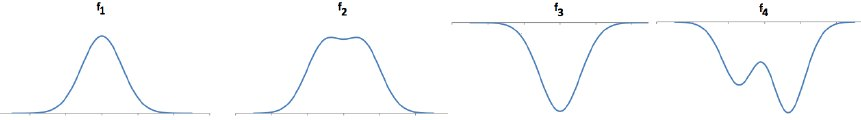Teaching Physics with the Physics Suite Edward F. Redish Problems Sorted by Type | Problems Sorted by Subject | Problems Sorted by Chapter in UP

Potential energy analogs for chemical reactions

A. You’ve been talking about the concept of energy for many years – since grade school. You’ve probably had chemistry and biology classes in which energy was a central element of the conversation. Now in physics we are slowly building the concept of a particular kind of energy – mechanical energy (kinetic and potential). What do you think energy is? How would you explain it to a non-scientific roommate? To a seven year old? (Not just “mechanical energy” – all energy.)

A variety of interesting behaviors can be described with different shapes of potential energy. Four qualitatively different PE shapes are shown in the figure below. In each figure, the horizontal axis is a position and the vertical axis is a potential energy. You can think of them as frictionless tracks with a small ball rolling on them. The energy they describe is like a gravitational potential energy of the ball.• The graph labeled f1 is a hill and represents an unstable equilibrium. A ball can sit at the top of this hill, but a small deviation from the exact center will lead to forces that will cause the ball to roll down the hill and convert the potential energy to kinetic.

• The graph labeled f2 is a hill with a dip and represents a metastable state. If a ball is sitting in the center of the dip at the top, a very small deviation will just oscillate back and forth a tiny bit. But if you provide the ball with just a bit more of kinetic energy, it will have enough energy to go up over the lip of the inner dip and roll down the long hill, gaining lots of kinetic energy in the process.

• The graph labeled f3 is a well. When the ball is at the bottom of the well, it is a bound state. You have to give the ball kinetic energy equal to the depth of well to get it out. If you do that, when it gets out, it would have 0 kinetic energy left, using it all up in climbing out of the well.

• The graph labeled f4 is a double well. Suppose the ball is at the bottom of the left well. Giving the ball a little kinetic energy -- enough to get it over the hump in the middle -- would let it roll into the deeper well, gaining a lot of kinetic energy in the process. You can go from one bound state to a more deeply bound one and get some additional energy (in this case kinetic) as a result.

Some of these situations are analogous to the situation of chemical bonding. Consider the following examples and discuss which if any of the potential energy shapes provide a useful analogy. Explain why you think so. (The energy going into or coming out of a chemical reaction may be in the form of kinetic energy -- seen as heat -- or in terms of electromagnetic energy -- a photon.)

B. Two moles hydrogen atoms may interact and form an H2 molecule. When they do so, they release 103 Cal (kcal).

C. Decomposing one mole of water molecules (H2O) into its component atoms requires the input of 220 Cal.

D. The decomposition of one mole of water molecules into H2 and O2 molecules requires the input of 113 Cal.

E. The phosphorus in the head of a match burns in air, giving off energy when you strike the match.